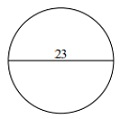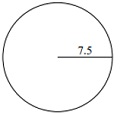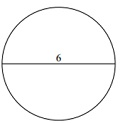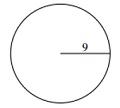# Circumference of a Circle Online Quiz

Following quiz provides Multiple Choice Questions (MCQs) related to Circumference of a Circle. You will have to read all the given answers and click over the correct answer. If you are not sure about the answer then you can check the answer using Show Answer button. You can use Next Quiz button to check new set of questions in the quiz.Q 1 - Find the circumference of following circle. Take π = 3.14### Explanation

Step 1:

Given diameter = 23 ; radius r = 23/2 = 11.5 units

Step 2:

Circumference of Circle = 2πr = 2(3.14)11.5 = 72.22 units

Q 2 - Find the circumference of following circle. Take π = 3.14### Explanation

Step 1:

Given diameter = 15 ; radius r = 23/2 = 7.5 units

Step 2:

Circumference of Circle = 2πr = 2(3.14)7.5 = 47.1 units

Q 3 - Find the circumference of following circle. Take π = 3.14### Explanation

Step 1:

Given radius r = 7.5 units

Step 2:

Circumference of Circle = 2πr = 2(3.14)7.5 = 47.1 units

Q 4 - Find the circumference of following circle. Take π = 3.14### Explanation

Step 1:

Given diameter = 6 ; radius r = 6/2 = 3 units

Step 2:

Circumference of Circle = 2πr = 2(3.14)3 = 18.84 units

Q 5 - Find the circumference of following circle. Take π = 3.14### Explanation

Step 1:

Given radius r = 8.5 units

Step 2:

Circumference of Circle = 2πr = 2(3.14)8.5 = 53.38 units

Q 6 - Find the circumference of following circle. Take π = 3.14### Explanation

Step 1:

Given radius r = 9 units

Step 2:

Circumference of Circle = 2πr = 2(3.14)9 = 56.52 units

Q 7 - Find the circumference of following circle. Take π = 3.14### Explanation

Step 1:

Given diameter = 19 ; radius r = 19/2 = 9.5 units

Step 2:

Circumference of Circle = 2πr = 2(3.14)9.5 = 59.66 units

Q 8 - Find the circumference of following circle. Take π = 3.14### Explanation

Step 1:

Given diameter = 29 ; radius r = 29/2 = 14.5 units

Step 2:

Circumference of Circle = 2πr = 2(3.14)14.5 = 91.06 units

Q 9 - Find the circumference of following circle. Take π = 3.14### Explanation

Step 1:

Given diameter = 1 ; radius r = 1/2 = 0.5 unit

Step 2:

Circumference of Circle = 2πr = 2(3.14)0.5 = 3.14 units

Q 10 - Find the circumference of following circle. Take π = 3.14### Explanation

Step 1:

Given radius r = 6 units

Step 2:

Circumference of Circle = 2πr = 2(3.14)6 = 37.68 units

circumference_of_a_circle.htm